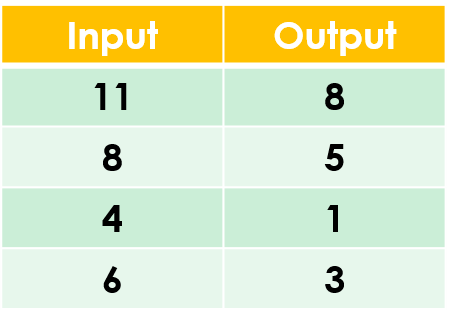1
visibility

Find the rule from the table below:• A

Subtract 5

• B

Subtract 3

The correct answer is "Subtract 3".

Please Note:
Subtraction is the operation that decreases a number by taking away from it.

Solution:
To find the correct rule, we consider the given table.

We observe that the given rule makes each number decrease. This implies subtraction.

We try subtracting 3 from each input number:

11 - 3 = 8
8 - 3 = 5
4 - 3 = 1
6 - 3 = 3

On subtracting 3 from the input numbers, we get the corresponding output numbers.

So, the rule is “subtract 3”.